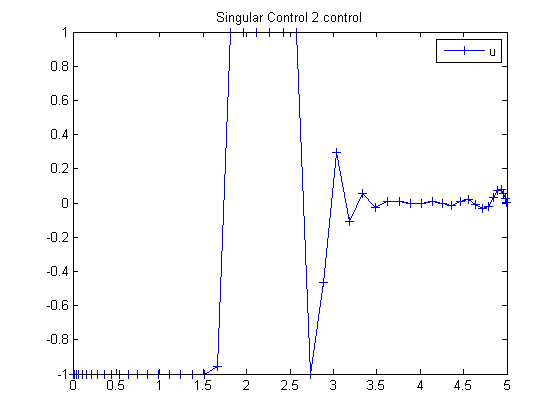102  Singular Control 2

ITERATIVE DYNAMIC PROGRAMMING, REIN LUUS

10.2.2 Example 2

CHAPMAN & HALL/CRC Monographs and Surveys in Pure and Applied Mathematics

102.1  Problem Formulation

Find u over t in [0; 5 ] to minimize

 J = x3(tF)

subject to:

 dx1 dt
= x2
 dx2 dt
= u
 dx3 dt
= x12

The initial condition are:

 x(0) = [0  1  0]
 −1 <= u <= 1

Reference: 

102.2  Problem setup

toms t
p = tomPhase('p', t, 0, 5, 50);
setPhase(p);

tomStates x1 x2 x3
tomControls u

% Initial guess
x0 = {icollocate({x1 == 0; x2 == 1; x3 == 0})
collocate(u == 0)};

% Box constraints
cbox = {-1 <= collocate(u) <= 1};

% Boundary constraints
cbnd = initial({x1 == 0; x2 == 1; x3 == 0});

% ODEs and path constraints
ceq = collocate({dot(x1) == x2
dot(x2) == u; dot(x3) == x1.^2});

% Objective
objective = final(x3);

102.3  Solve the problem

options = struct;
options.name = 'Singular Control 2';
solution = ezsolve(objective, {cbox, cbnd, ceq}, x0, options);
t  = subs(collocate(t),solution);
u  = subs(collocate(u),solution);
Problem type appears to be: lpcon
Starting numeric solver
===== * * * =================================================================== * * *
TOMLAB - Tomlab Optimization Inc. Development license  999001. Valid to 2011-02-05
=====================================================================================
Problem: ---  1: Singular Control 2             f_k       0.268336059478540890
sum(|constr|)      0.000000004483254193
f(x_k) + sum(|constr|)      0.268336063961795100
f(x_0)      0.000000000000000000

Solver: snopt.  EXIT=0.  INFORM=1.
SNOPT 7.2-5 NLP code
Optimality conditions satisfied

FuncEv    1 ConstrEv  107 ConJacEv  107 Iter  100 MinorIter  353
CPU time: 0.843750 sec. Elapsed time: 0.875000 sec.

102.4  Plot result

figure(1)
plot(t,u,'+-');
legend('u');
title('Singular Control 2 control');